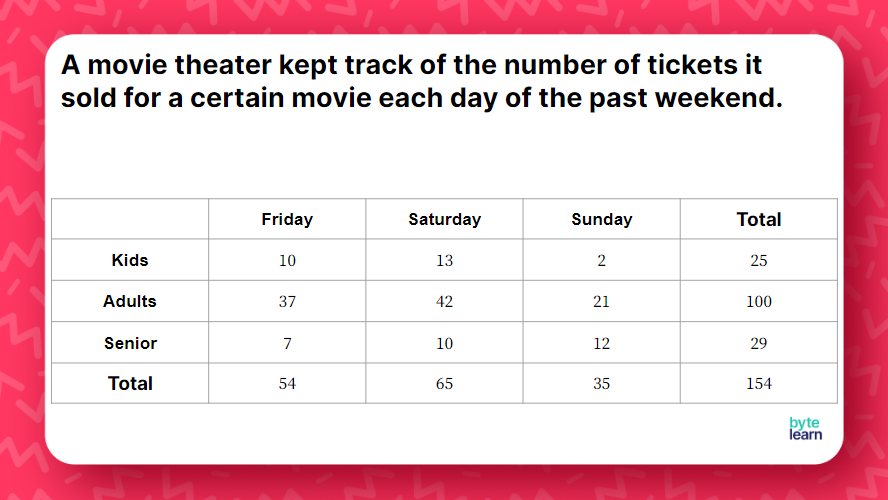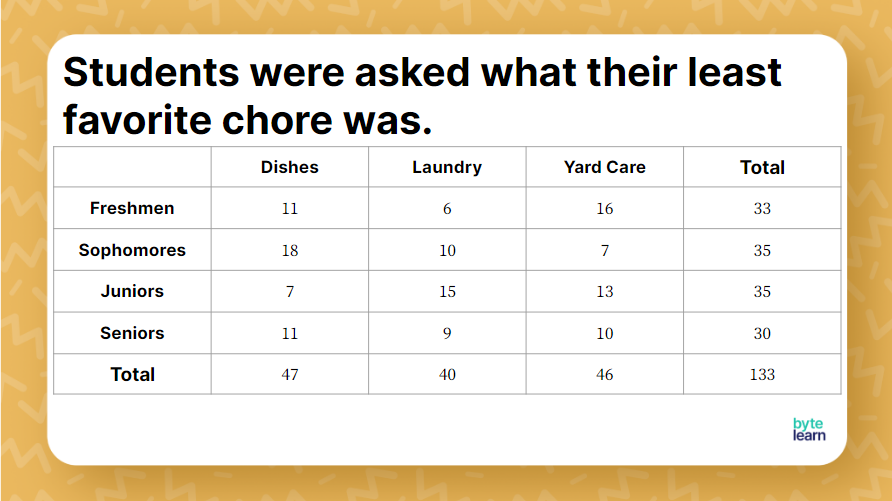ResourcesTestimonials
Plans
ResourcesTestimonials
PlansLesson plan

# Relative Frequency Lesson Plan

## Overview

In this lesson, students will learn how to calculate relative frequency. Students will begin by reviewing two-way tables. Then, students will work with peers to calculate the relative frequency for various two-way tables. You can expect this lesson with additional practice to take one 45-minute class period.

Statistics
8.SP.A.4

## Objective

Students will be able to calculate relative frequency as a fraction or percent.

## Materials

• Teacher slideshow
• Student Resource Sheet
• Online Practice

## How to Teach Relative Frequency

### Warm-up

In order to teach relative frequency, it is important for students to show they understand how to read two-way tables. You can give students a copy of the student resource sheet so they do not have to redraw each two-way table.

To start the lesson, allow students a couple of minutes to answer the questions that are given on slide one, which is the first example on their sheet.Students should be able to identify the values that correspond to each question. To extend on the questions that are already there, consider including questions like, “How many 6th graders preferred math?” You could also ask students to write down at least 3 things they can see from the table.

### Introduction to relative frequency

With the help of the example from the warm-up and some additional questions, students will begin to understand the concept of relative frequency.

The first two questions are similar, and some students are likely to say the answers are the same. Students are generally able to identify the numerators; however, they can struggle with the denominators. “Fraction of 6th graders” indicates that it refers to the total number of 6th graders. “Fraction of students who preferred science” indicates that the total number of students was 25.

### Example 1

Using the same two-way table from the warm-up, explain the general concept of relative frequency. Students should understand that it can be written as a fraction, decimal, or percent. Students should also understand it compares a part to a whole (or total), and it will vary depending on the circumstances.

With these first examples, it is important for students to recognize how to determine which values are needed. Ask students to identify which values are relevant for the first statement. Students should share their thoughts with a partner or table group to explain which values they thought would be relevant and why. If students are not able to immediately recognize which values matter, it will be important to spend time to explain why.

### Writing as the fraction with words

It’s sometimes helpful for students to write the fraction with words:
\frac{\text{number of people surveyed from Canada}}{\text{total number of people surveyed}}

Repeat this process with the following two statements. In each case, allow students an opportunity to identify which values are relevant. Ideally, students will begin to also be able to identify which value should be the numerator and which should be the denominator.

### Example 2

With this next example, students will see that two-way tables can have more than 2 categories for the rows or columns. The relative frequency questions for this table are on the student resource sheet and include the following:

• What fraction of people went to the movie on Saturday?
• What percent of people were adults who saw the movie on Friday?
• What percent of people were kids who saw the movie on Sunday?
• What fraction of people were seniors who saw the movie on Saturday?Depending on students’ abilities, you can give them an opportunity to try all of the problems; however, you may need to walk through each relative frequency individually.

### Example 3

For this example, students have more possibilities in the two-way table. On their sheets, they’re given the following questions.

• What fraction of people surveyed were sophomores?
• What fraction of people surveyed were juniors whose least favorite chore was laundry?
• What percent of freshmen surveyed said their least favorite chore was dishes?
• What percent of people surveyed said their least favorite chore was yard care?### Reflection

At this point, it may be helpful to ask students to reflect on what they have learned. What strategies did they use that helped them understand the problems? What strategies did not work for them? Where did they struggle the most? Having students reflect on questions like this can help them build their metacognition to be more cognizant of their own learning.

## Relative Frequency Practice

After you’ve completed the examples with the whole class, it’s time for some independent practice! ByteLearn gives you access to tons of practice problems for calculating relative frequency. Check out the online practice and assign to your students for classwork and/or homework!Relative Frequency Practice
Problem 1 of 5
<p>A fitness club asks different types of athletes what time of day they prefer to exercise. </p><TableUIv2 data-props='{ "headers": [ { "value": "Type of Athlete" }, { "value": "Morning" }, { "value": "Afternoon" }, { "value": "Evening" }, { "value": "Total" } ], "borders": {}, "rows": [ [ { "value": "Runners", "dim": false, "highlight": false }, { "value": "12", "dim": false, "highlight": false }, { "value": "5", "dim": false, "highlight": false }, { "value": "8", "dim": false, "highlight": false }, { "value": "25", "dim": false, "highlight": false } ], [ { "value": "Bikers", "dim": false, "highlight": false }, { "value": "6", "dim": false, "highlight": false }, { "value": "14", "dim": false, "highlight": false }, { "value": "12", "dim": false, "highlight": false }, { "value": "32", "dim": false, "highlight": false } ], [ { "value": "Swimmers", "dim": false, "highlight": false }, { "value": "7", "dim": false, "highlight": false }, { "value": "11", "dim": false, "highlight": false }, { "value": "15", "dim": false, "highlight": false }, { "value": "33", "dim": false, "highlight": false } ], [ { "value": "Total", "dim": false, "highlight": false }, { "value": "25", "dim": false, "highlight": false }, { "value": "30", "dim": false, "highlight": false }, { "value": "35", "dim": false, "highlight": false }, { "value": "90", "dim": false, "highlight": false } ] ]}'></TableUIv2 ><p>What percent of the athletes are runners who prefer to exercise in the morning?</p>

View this practice